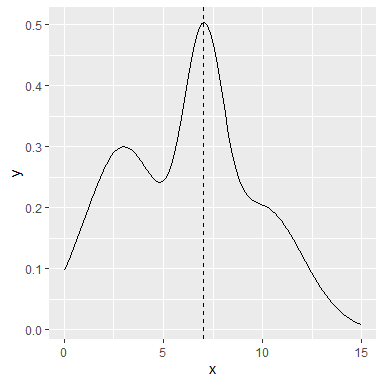# Function Maximization

## Simple Example

Bayesian Optimization seek the global maximum of any user defined function. As a simple example, let’s define a simple function:

library(ggplot2)
library(ParBayesianOptimization)
simpleFunction <- function(x) dnorm(x,3,2)*1.5 + dnorm(x,7,1) + dnorm(x,10,2)
maximized <- optim(8,simpleFunction,method = "L-BFGS-B",lower = 0, upper = 15,control = list(fnscale = -1))$par ggplot(data = data.frame(x=c(0,15)),aes(x=x)) + stat_function(fun = simpleFunction) + geom_vline(xintercept = maximized,linetype="dashed")We can see that this function is maximized around x~7.023. We can use bayesOpt to find the global maximum of this function. We just need to define the bounds, and the initial parameters we want to sample: bounds <- list(x=c(0,15)) initGrid <- data.frame(x=c(0,5,10)) Here, we run bayesOpt. The function begins by running simpleFunction 3 times, and then fits a Gaussian process to the results in a process called Kriging. We then calculate the x which maximizes our expected improvement, and run simpleFunction at this x. We then go through 1 more iteration of this: FUN <- function(x) list(Score = simpleFunction(x)) optObj <- bayesOpt( FUN = FUN , bounds = bounds , initGrid = initGrid , acq = "ei" , iters.n = 2 , gsPoints = 25 ) Let’s see how close the algorithm got to the global maximum: getBestPars(optObj) #>$x
#>  6.410781

The process is getting pretty close! We were only about 12% shy of the global optimum:

simpleFunction(7.023)/simpleFunction(getBestPars(optObj)$x) #>  1.132927 Let’s run the process for a little longer: optObj <- addIterations(optObj,iters.n=2,verbose=0) simpleFunction(7.023)/simpleFunction(getBestPars(optObj)$x)
#>  1.000454

We have now found an x very close to the global optimum.